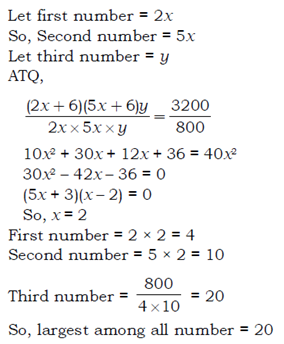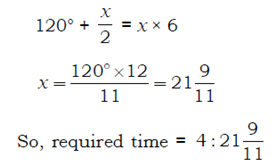# SSC CGL 2018 Practice Test Papers | Quantitative Aptitude (Day-30)

Dear Aspirants, Here we have given the Important SSC CGL Exam 2018 Practice Test Papers. Candidates those who are preparing for SSC CGL 2018 can practice these questions to get more confidence to Crack SSC CGL 2018 Examination.

[WpProQuiz 3200]

Click “Start Quiz” to attend these Questions and view Explanation

1) The average age of six persons in a group is 8 years. The average age of three persons of this group is 9 years. What is the average age of other three persons in the group?

a) 5

b) 7

c) 8

d) 10

2) The average weight of 45 boys in a school is 50 kg. If the weight of 5 girl’s student is added, the average is reduced by half a kilogram. What is the average weight of the girl students?

a) 45 kg

b) 44.5 kg

c) 46.5 kg

d) 45.5 kg

3) The product of three integers is 800. The ratio of first and second is 2 : 5. If 6 is added both the first and the second and third remains unaltered, the product becomes 3200. The greatest of the three integers is

a) 80

b) 40

c) 20

d) 10

4) At what time between 4 O’clock and 5 O’clock, will the hands of a clock be together?

a) 4: 21 9/11

b) 4 : 19 1/12

c) 4: 23 3/17

d) 4: 27 1/7

5) Simplify: 560+23-7×39/21

a) 570

b) 650

c) 420

d) 530

Direction (6-10): The following table shows production (in thousands) of two types (P and Q) of vehicles by a factory over the years 2009 to 2014.

 Years Type P Type Q 2009 100 175 2010 125 150 2011 200 125 2012 225 175 2013 275 175 2014 275 225

6) The total production of Type P vehicles in the years 2009 and 2011 is what percent of total production of Type Q vehicles in years 2010 and 2014?

a) 81.25%

b) 69.25%

c) 80%

d) 75%

7) In how many of the given years, was the production of Type P vehicles of the company more than the average production of these type vehicles in the given years?

a) 4

b) 5

c) 3

d) 2

8) The production of Type Q vehicles in 2010 was approximately what percent of Type P vehicles in 2014 ?

a) 75%

b) 54.5%

c) 45.5%

d) 60%

9) Approximate percentage decrease in production of Type Q vehicles from years 2010 to 2011 is

a) 16.7%

b) 20.3%

c) 10%

d) 12.5%

10) The ratio of total production of Type P vehicles to total production of Type Q vehicles Over the years is –

a) 50: 51

b) 48: 41

c) 35: 37

d) 42: 51

Age of six persons= 6×8=48

Age of three persons=3×9=27

Age of other three persons=48-27=21

Average age =21/3=7 years

Average weight of 45 boys in a school = 50 kg

Average weight of (45 boys + 5 girls) in that school = 49.5 kg

So, average weight of 5 girls

= (50 x 49.5- 45x 50)/5

= 45 kg560+23-7×39/21= 570

Required percentage = (100 +200)/ (150+225) x100=80%

Average of production in 2009= (100+175)/2=137.5=>137.5>100

Average of production in 2010= (125+150)/2=137.5=>137.5> 125

Average of production in 2011= (200+125)/2=162.5=>162.5<200

Average of production in 2012= (225+175)/2=200=> 200<225

Average of production in 2013= (275+175)/2=225=> 225<275

Average of production in 2014= (275+225)/2=250=> 250<275

Required percentage =150/275 × 100

= 54.5%

Required percentage = (150 -125)/150× 100

= 16.7%